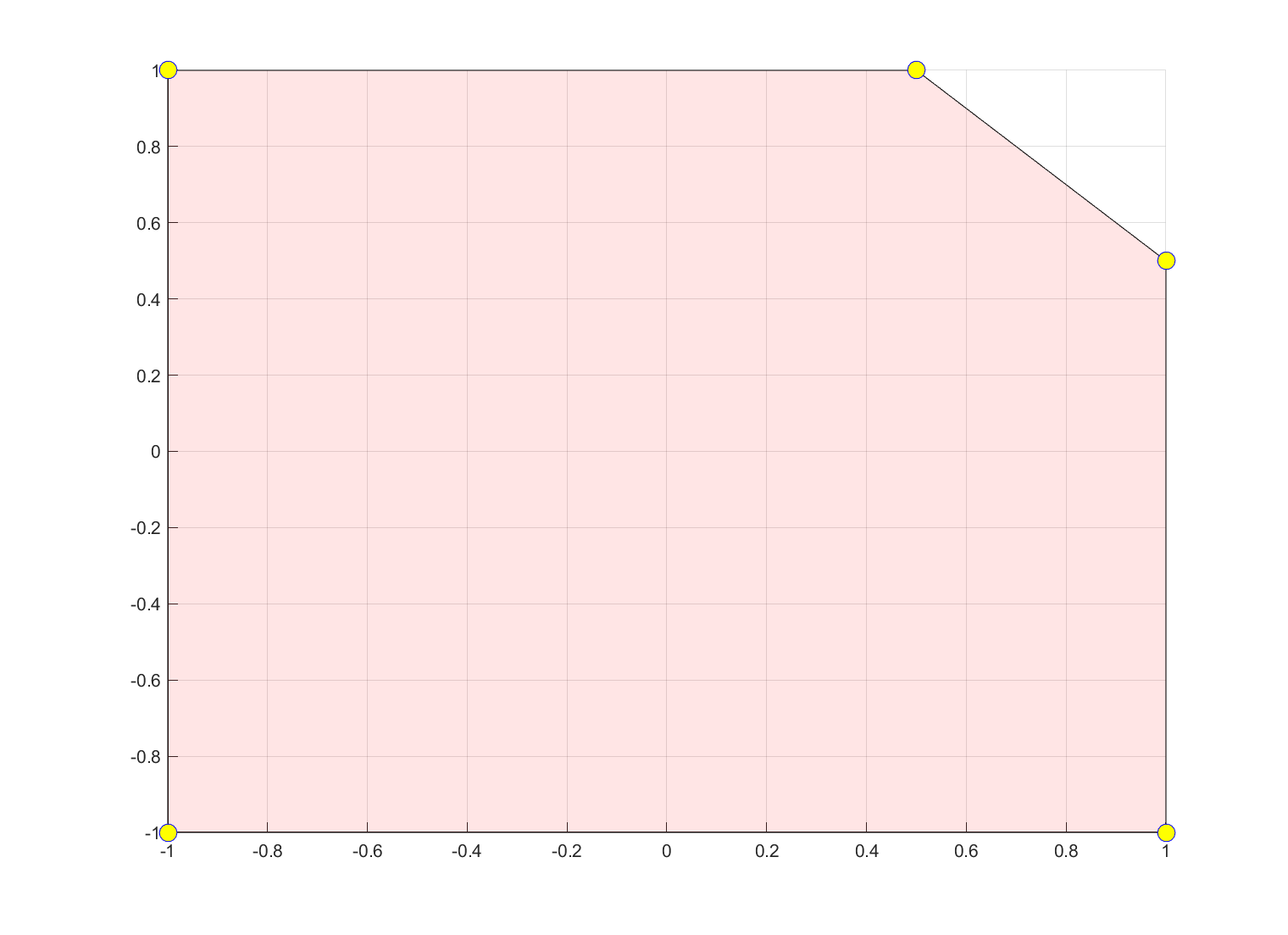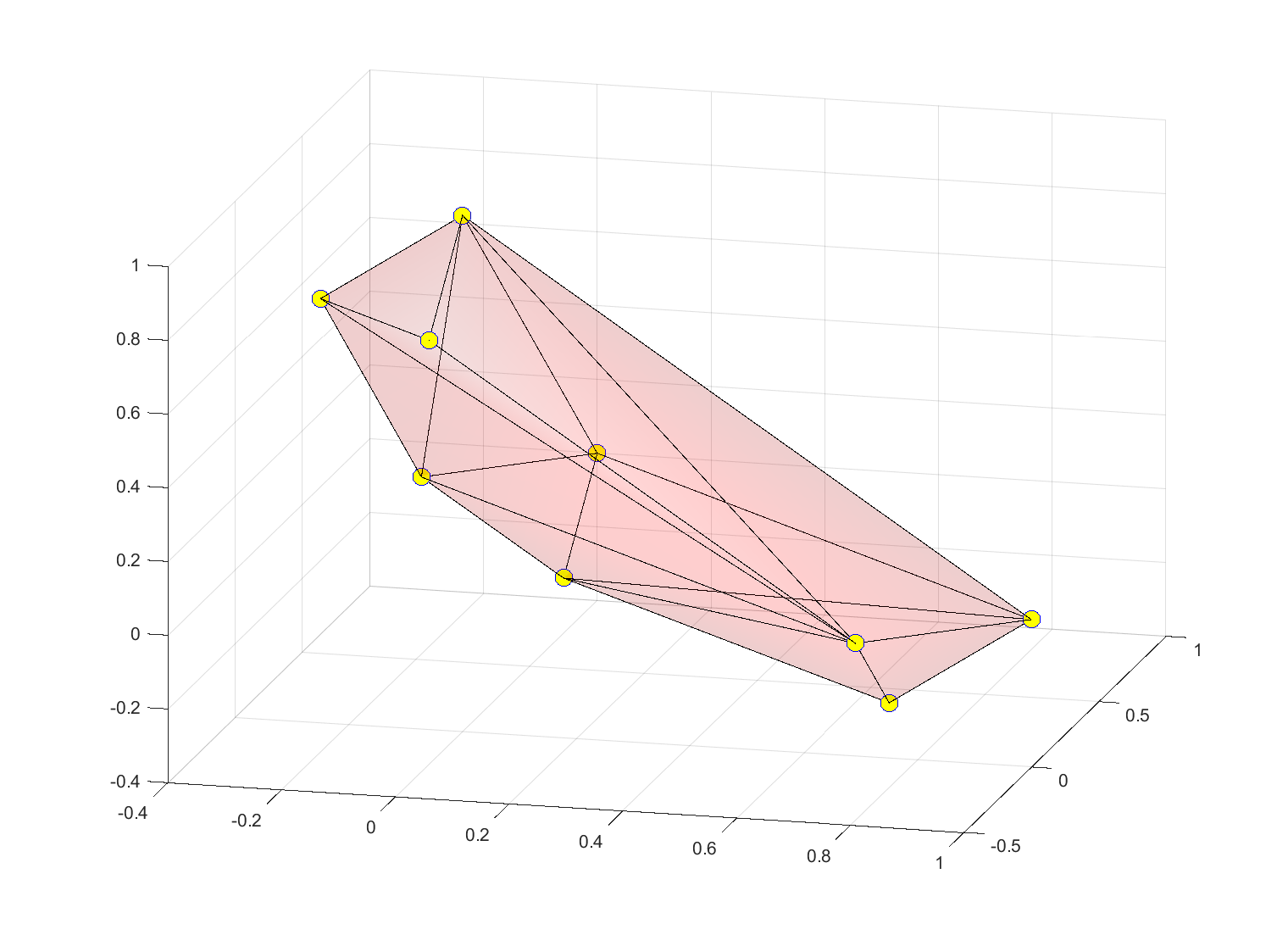# vertex

Tags:

Updated:

vertex computes the vertices of a polytopic constraint.

## Syntax

V = vertex(Constraint,[x])


## Example

The typical use is as follows

x = sdpvar(2,1);
B = [-1 <= x <= 1, sum(x) <= 3/2];
clf
hold on;grid on
v = vertex(B,x);
m = plot(v(1,:),v(2,:),'bo');
set(m,'Markersize',10);
set(m,'Markerface','yellow');Models often live in a higher-dimensional space than expected due to internal modelling. For instance, the following polytope in 3D is represented in a 6D space in order to represent the absolute value using epigraph variables. To find the vertices of the polytope in our original space, we give a second argument to explain that we only want the (convex hull of the) vertices projected onto our original space.

x = sdpvar(3,1);
P = [-1 <= x <= 1, sum(abs(x)) <= 1,sum(x)>=.5]
clf